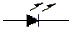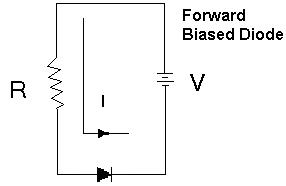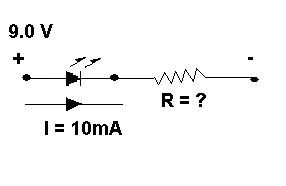#### LEDs – Light Emitting Diodes

The LED is often used in circuits as an indicator lamp to show if a system is ON or OFF.
The schematic symbol for a light emitting diode isLEDs use very low voltage (about 1.5V).  Higher voltages will damage the LED.
To protect the LED in a circuit with voltages higher than 1.5 V, we use a resistor in series with the LED.The above circuit shows a forward biased LED with a resistor protecting it from burning out.

A typical LED will be safe when no more than 10 mA (0.01 A) of current flow through it and will have no more than 2.0 V across it.
We can calculate the size of a resistor required to safely connect a LED.
Example:
What size resistor do we need to safely connect a 10mA LED to a 9V battery?
Look at the circuit below:Solution:

The LED will safely absorb 2.0 Volts.
Therefore there are 7.0 V left to be absorbed by the resistor.
(9.0 V– 2.0 V = 7.0 V).
The current is the same throughout the circuit 10 mA = 0.01A
--- this is a series circuit.

Using Ohm’s LAW we can calculate the resistance of the resistor.

### R = V/I = 7.0 V / 0.01 A = 700 ohms

The colour code for this resistor is:
violet, black, brown
Exercise:

1. What value of resistance is needed to protect a LED connected to the following voltage sources?
1. 10 V
2. 5 V
3. 2.5 V

2. What are the respective colour codes for each of the resistors calculated in exercise #1?

Solutions:
Problem 1
• Voltage across LED = 2.0V
• Voltage across Resistor = 10.0V – 2.0V = 8.0V
• Current through Diode & Resistor = 10mA =0.01A

#### R = V/I = 8.0V/0.01A = 800 ohms

• Voltage across LED = 2.0V
• Voltage across Resistor = 5.0V – 2.0V = 3.0V
• Current through Diode & Resistor = 10mA =0.01A

### R = V/I = 5.0V/0.01A = 300 ohm

• Voltage across LED = 2.0V
• Voltage across Resistor = 2.5V – 2.0V = 0.5V
• Current through Diode & Resistor = 10mA =0.01A

Problem 2

### R = V/I = 0.5V/0.01A = 50 ohm

• 800 ohms  = gray, black, brown
• 300 ohms = red, black, brown
•  50 ohms = green, black, black